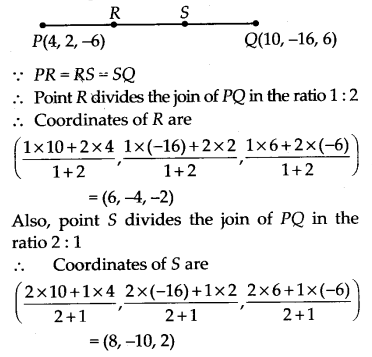NCERT Solutions for Class 11 Maths Chapter 12 Introduction to Three Dimensional Geometry Ex 12.3

# NCERT Solutions for Class 11 Maths Chapter 12 Introduction to Three Dimensional Geometry Ex 12.3

## NCERT Solutions for Class 11 Maths Chapter 12 Introduction to Three Dimensional Geometry Ex 12.3

NCERT Solutions for Class 11 Maths Chapter 12 Introduction to Three Dimensional Geometry Ex 12.3 are the part of NCERT Solutions for Class 11 Maths. Here you can find the NCERT Solutions for Class 11 Maths Chapter 12 Introduction to Three Dimensional Geometry Ex 12.3.

### Ex 12.3 Class 11 Maths Question 1.

Find the coordinates of the point which divides the line segment joining the points (-2, 3, 5) and (1, -4, 6) in the ratio
(i) 2 : 3 internally,
(ii) 2 : 3 externally

Solution:
(i) Let P(x, y, z) be any point which divides the line segment joining the points A(-2, 3, 5) and B(1, -4, 6) in the ratio 2 : 3 internally.

(ii) Let P(x, y, z) be any point which divides the line segment joining the points A(-2, 3, 5) and B(1, -4, 6) in the ratio 2 : 3 externally. Then

### Ex 12.3 Class 11 Maths Question 2.

Given that P(3, 2, -4), Q(5, 4, -6) and R(9, 8, -10) are collinear. Find the ratio in which Q divides PR.

Solution:
Let Q(5, 4, -6) divides the line segment joining the points P(3, 2, -4) and R(9, 8, -10) in the ratio k : 1 internally.

### Ex 12.3 Class 11 Maths Question 3.

Find the ratio in which the YZ-plane divides the line segment formed by joining the points (-2, 4, 7) and (3, -5, 8).

Solution:
Let the ratio in which the YZ-plane divides the line segment formed by joining the points A(-2, 4, 7) and B(3, -5, 8) at a point C be k : 1.

### Ex 12.3 Class 11 Maths Question 4.

Using section formula, show that the points A(2, -3, 4), B(-1, 2, 1) and C(0, 1/3, 2) are collinear.

Solution:
Let the points A(2, -3, 4), B(-1, 2, 1) and C(0, 1/3, 2) be the given points. Let the point P divides AB in the ratio k : 1. Then, the coordinates of P are [(−k+2)/(k+1), (2k3)/(k+1), (k+4)/(k+1)]
Let us examine whether for some value of k, the point P coincides with point C.

AB internally in the ratio 2 : 1. Hence A, B, C are collinear.

### Ex 12.3 Class 11 Maths Question 5.

Find the coordinates of the points which trisect the line segment joining the points P(4, 2, -6) and Q(10, -16, 6).

Solution:
Let R and S be two points which trisect the line segment joining the points P(4, 2, -6) and Q(10, -16, 6).You can also like these:

NCERT Solutions for Maths Class 9

NCERT Solutions for Maths Class 10

NCERT Solutions for Maths Class 12